# Math in Focus Grade 3 Chapter 4 Practice 3 Answer Key Subtraction with Regrouping in Ones, Tens, Hundreds, and Thousands

Go through the Math in Focus Grade 3 Workbook Answer Key Chapter 4 Practice 3 Subtraction with Regrouping in Ones, Tens, Hundreds, and Thousands to finish your assignments.

## Math in Focus Grade 3 Chapter 4 Practice 3 Answer Key Subtraction with Regrouping in Ones, Tens, Hundreds, and Thousands

Subtract. Fill in the blanks.

Question 1.
Example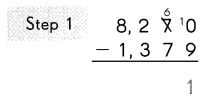Subtract the ones.
9 ones cannot be subtracted from 0 ones.
So, regroup the tens and ones.
7 tens 0 ones
= __ tens ___ ones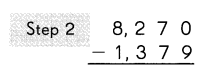Subtract the tens.
7 tens cannot be subtracted from 6 tens.
So, regroup the hundreds and tens.
2 hundreds 6 tens
= __ hundred ___ tens
Subtract the hundreds.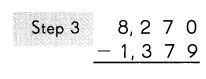3 hundreds cannot be subtracted from
___ hundred.
So, regroup the thousands and hundreds.
8 thousands 1 hundred
= ___ thousands ___ hundreds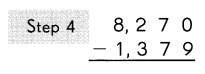Subtract the thousands.

8270 – 1379 = 6891.

Explanation:Subtract the ones.
9 ones cannot be subtracted from 0 ones.
So, regroup the tens and ones.
7 tens 0 ones
= 6 tens 10 ones.Subtract the tens.
7 tens cannot be subtracted from 6 tens.
So, regroup the hundreds and tens.
2 hundreds 6 tens
= 1 hundred 16 tens.
Subtract the hundreds.3 hundreds cannot be subtracted from 2 hundred.
So, regroup the thousands and hundreds.
8 thousands 1 hundred
= 7 thousands 11 hundredsSubtract the thousands.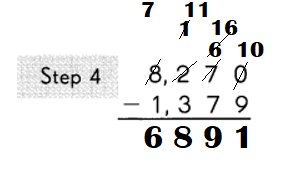Subtract. Fill in the blanks.

Question 2.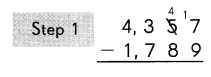Subtract the ones.
9 ones cannot be subtracted from 7 ones.
So, regroup the tens and ones.
5 tens 7 ones
= ___________ tens ___________ ones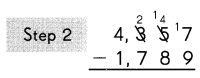Subtract the tens.
8 tens cannot be subtracted from ___________ tens.
So, regroup the hundreds and tens.
3 hundreds 4 tens
= __________ hundreds __________ tens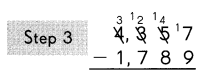Subtract the hundreds.
7 hundreds cannot be subtracted from
___________ hundreds.
So, regroup the thousands and hundreds.
4 thousands 2 hundreds.
= ____ thousands __ hundreds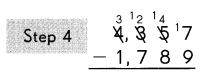Subtract the thousands.

4357 – 1789 = 2568.

Explanation:Subtract the ones.
9 ones cannot be subtracted from 7 ones.
So, regroup the tens and ones.
5 tens 7 ones
= 4 tens 17 ones.Subtract the tens.
8 tens cannot be subtracted from 4 tens.
So, regroup the hundreds and tens.
3 hundreds 4 tens
= 2 hundreds 14 tens.Subtract the hundreds.
7 hundreds cannot be subtracted from
2 hundreds.
So, regroup the thousands and hundreds.
4 thousands 2 hundreds.
= 3 thousands 12 hundreds.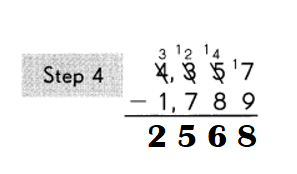Subtract. Regroup when needed.

Question 3.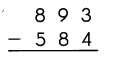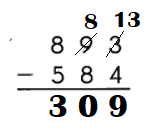Explanation:
Step:1
Subtract the ones.
3 ones cannot be subtracted from 4 ones.
So, regroup the tens and ones.
9 tens 3 ones
= 8 tens 13 ones.

Step:2:
Subtract the tens.

Step:3
Subtract the hundreds.

Question 4.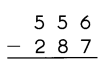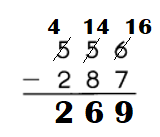Explanation:
Step:1
Subtract the ones.
7 ones cannot be subtracted from 6 ones.
So, regroup the tens and ones.
5 tens 6 ones
= 4 tens 16 ones.

Step:2:
Subtract the tens.
4 tens cannot be subtracted from 8 tens.
So, regroup the hundreds and tens.
5 hundreds 4 tens
= 4 hundreds 14 tens.

Step:3
Subtract the hundreds.

Question 5.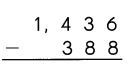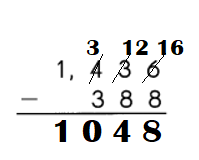Explanation:
Step:1
Subtract the ones.
8 ones cannot be subtracted from 6 ones.
So, regroup the tens and ones.
3 tens 6 ones
= 2 tens 16 ones.

Step:2:
Subtract the tens.
8 tens cannot be subtracted from 2 tens.
So, regroup the hundreds and tens.
4 hundreds 2 tens
= 3 hundreds 12 tens.

Step:3
Subtract the hundreds.

Question 6.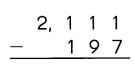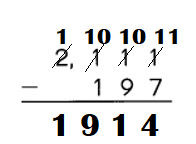Explanation:
Step:1
Subtract the ones.
7 ones cannot be subtracted from 1 ones.
So, regroup the tens and ones.
1 tens 1 ones
= 10 tens 11 ones.

Step:2:
Subtract the tens.
9 tens cannot be subtracted from 0 tens.
So, regroup the hundreds and tens.
1 hundreds 0 tens
= 0 hundreds 10 tens.

Step:3
Subtract the hundreds.
1 hundreds cannot be subtracted from
0 hundreds.
So, regroup the thousands and hundreds.
2 thousands 0 hundreds.
= 1 thousands 10 hundreds.

Step:4
Subtract the thousands.

Question 7.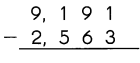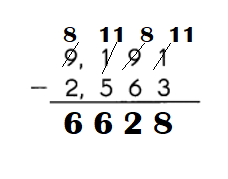Explanation:
Step:1
Subtract the ones.
3 ones cannot be subtracted from 1 ones.
So, regroup the tens and ones.
9 tens 1 ones
= 8 tens 11 ones.

Step:2:
Subtract the tens.

Step:3
Subtract the hundreds.
5 hundreds cannot be subtracted from 1 hundreds.
So, regroup the thousands and hundreds.
9 thousands 1 hundreds.
= 8 thousands 11 hundreds.

Step:4
Subtract the thousands.

Question 8.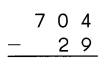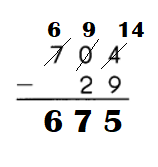Explanation:
Step:1
Subtract the ones.
9 ones cannot be subtracted from 4 ones.
So, regroup the tens and ones.
0 tens 4 ones
=  tens 14 ones.

Step:2:
Subtract the tens.
2 tens cannot be subtracted from 0 tens.
So, regroup the hundreds and tens.
7 hundreds 0 tens
= 6 hundreds 9 tens.

Step:3
Subtract the hundreds.

Question 9.
Color the answers from above to find the path to the present.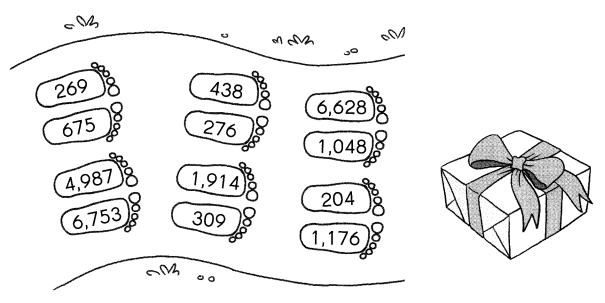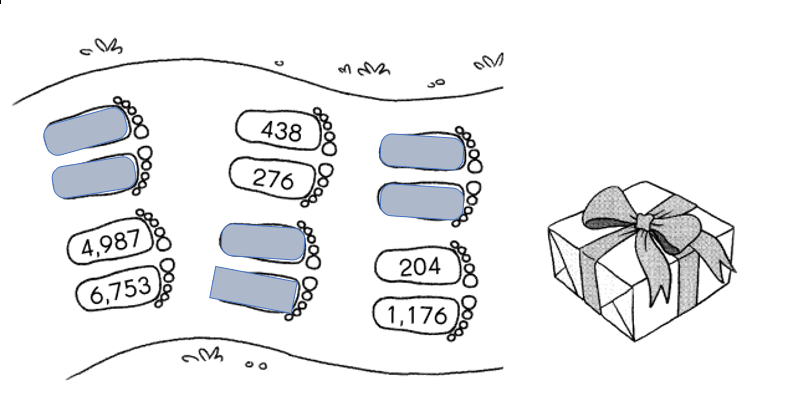Explanation:
8270 – 1379 = 6891.
4357 – 1789 = 2568.Subtract. Regroup when needed.
Example
3,852 – 1,621 = 2,231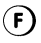Question 10.
7,162 – 5,002 = ___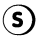7,162 – 5,002 = 2160.Explanation:
7,162 – 5,002 = 2160.

Question 11.
7,156 – 43 = ___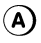7,156 – 43 = 7113.Explanation:
7,156 – 43 = 7113.

Question 12.
3,696 – 2,475 = ___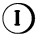3,696 – 2,475 = 1221.Explanation:
3,696 – 2,475 = 1221.

Question 13.
7,342 – 2,502 = ___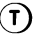7,342 – 2,502 = 4840.Explanation:
7,342 – 2,502 = 4840.

Question 14.
8,513 – 566 = ___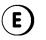8,513 – 566 = 7947.Explanation:
8,513 – 566 = 7947.

Question 15.
6,707 – 1,125 = ___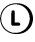6,707 – 1,125 = 5582.Explanation:
6,707 – 1,125 = 5582.

Question 16.
2,152 – 1,648 = ___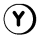2,152 – 1,648 = 504.Explanation:
2,152 – 1,648 = 504.

Question 17.
5,261 – 85 = ___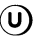5,261 – 85 = 5176.Explanation:
5,261 – 85 = 5176.

Question 18.
9,133 – 7,269 = ___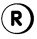9,133 – 7,269 = 1864.Explanation:
9,133 – 7,269 = 1864.

Question 19.
3,087 – 1,779 = ___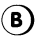3,087 – 1,779 = 1308.Explanation:
3,087 – 1,779 = 1308.

Question 20.
7,965 – 978 = ___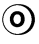7,965 – 978 = 6987.Explanation:
7,965 – 978 = 6987.

Write the corresponding letters from Exercise 11 to 20 to find the name of this national treasure.
Question 21.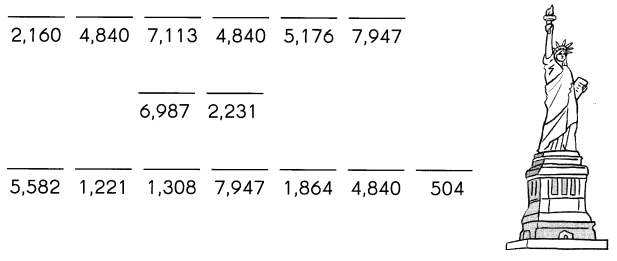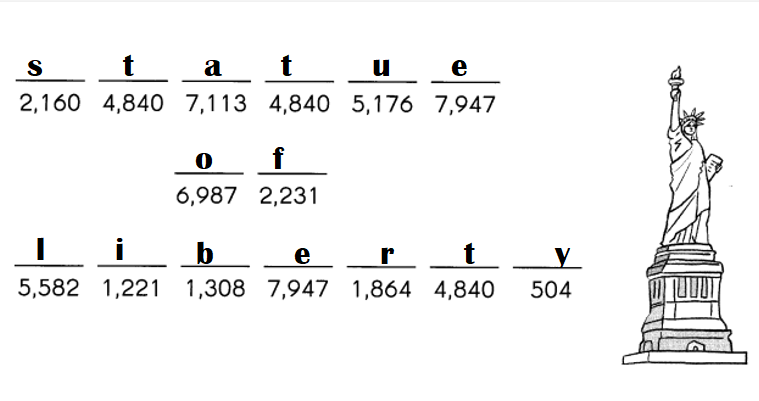Explanation:
3,852 – 1,621 = 2,2317,162 – 5,002 = 2160.7,156 – 43 = 7113.3,696 – 2,475 = 1221.7,342 – 2,502 = 4840.8,513 – 566 = 7947.6,707 – 1,125 = 5582.2,152 – 1,648 = 504.5,261 – 85 = 5176.9,133 – 7,269 = 1864.3,087 – 1,779 = 1308.7,965 – 978 = 6987.Question 22.
Where is this national treasure located?
____ __________